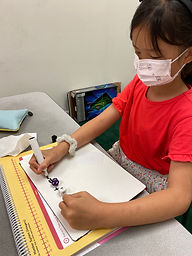## Ms. Kylie

### Target 1​

###### Lesson Type:

Continuation

Geometry

:

Shape

Measure, draw, and classify angles.

###### 1:

Accurately measure angles.

###### 2:

Understand that an angle is the union of two rays with a common endpoint (vertex).

###### 3:

Understand that angles are measured in degrees (from 0 to 360).

###### 4:

Understand that angles can be composed or decomposed, using addition and subtraction.

4th

###### Vocabulary:

Protractor, Degrees, Compose, Decompose

Activities:

-Student measured different angles using a protractor to find the degrees. Then used the answer to each problem to color a picture according to the color given.

-Student composed two angles to find the measurement of angle x. Addition was used to find the measurement of angle x.

-Student decomposed angles to find the missing angle measurement by finding the value of angle x.### Home Exploration

###### Guiding Questions:## Absent Students:

### Target 2

:

###### 1:

Understand that quantities having a value of more than zero are positive and that quantities having a value of less than zero are negative.

###### 2:

Understand that zero is neither positive nor negative.

###### 3:

Understand that integers get smaller in value as they move left in order, and larger as they move right in order.

5th

###### Vocabulary:

Positive, Negative

Activities:

-Student used a number line to add and subtract positive and negative numbers. The student rolled two dice to create an equation to add or subtract positive and negative numbers.### Home Exploration

###### Guiding Questions:### Target 3

:

###### 1:

Identify the correct arithmetic processes based on the information presented in word problems.

###### 2:

Find the key words in word problems that indicate the correct arithmetic process to use: Addition: add, altogether, both, combined, in all, plus, sum, total; Subtraction: difference, fewer, how many/much more, left, less, minus, remains; Multiplication: times, product, each, twice; and Division: how many in each, divided by, quotient, goes into, split evenly.

###### 3:

Find and use the needed information in a word problem to solve.

3rd

###### Vocabulary:

CUBES, Multi-Step Word Problem

Activities:

-Student used the strategy called CUBES (Circle numbers, Underline the question, Box important words, Eliminate words, Solve) to solve multi-step word problems for addition and subtraction.

-Students used the strategy to solve the word problem in steps.### Home Exploration